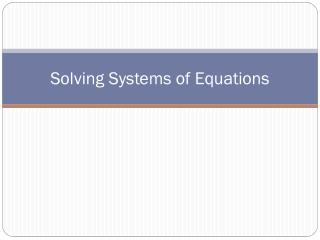DownloadDownload PresentationSolving Systems of Equations

Solving Systems of Equations

Download PresentationSolving Systems of Equations

- - - - - - - - - - - - - - - - - - - - - - - - - - - E N D - - - - - - - - - - - - - - - - - - - - - - - - - - -
Presentation Transcript

1. Solving Systems of Equations

2. Vocabulary • System of Equations: a set of equations with the same unknowns • Consistent: a system of equations with at least one ordered pair that satisfies both equations. • Dependent: a system of equations that has an infinite number of solutions; (lines are the same) • Inconsistent: a system of equations with no solution • Independent: a system of equations with exactly one solution • Solution to a system of equations: a point that makes both equations true

3. Different Methods for Solving Systems • Elimination Method: adding or subtracting the equations in the systems together so that one of the variables is eliminated; multiplication might be necessary first • Substitution Method: Solving one of a pair of equations for one of the variables and substituting that into the other equation • Graphing Method: Solving a system by graphing equations on the same coordinate plane and finding the point of intersection.

4. Substitution Method STEPS: • Solve one of the equations for one of the variables • Substitute, or replace, the resulting expression into the other equation for the solved variable • Solve the equation for the second variable • Substitute the found variable into either of the original equations to find the value of the other variable.

5. How do I write the solution? • If both values are found, the answer is written as an ordered pair • If the resulting solution is a true statement, like 9=9, the system has an infinite number of solutions • If the resulting solution is an untrue statement, like 0=9, then the system has no solution

6. Example 1 • Solve the following system by substitution

7. Example 2 • Solve the following system using substitution:

8. Example 3 • Solve the following system by substitution

9. Elimination Method STEPS: • If needed, multiply each term of the equation by the same number in order to get one pair of variables that are opposite signs but the same coefficient. • Add or subtract the two equations to eliminate one of the variables • Solve the equation for the second variable • Substitute the found value into either of the original equations to find the value of the other variable

10. Example 4 • Solve the following system using elimination

11. Example 5 • Solve the following system using elimination:

12. Example 6 • Solve the following system using elimination

13. Applications-Ex 1 • Ticket sales to the local gaming convention, PlayerCon, are on the rise! Sales of 2-day tickets brought in \$188,100. A total of 6,600 tickets were sold. Adult 2-day tickets cost \$36.00 and children’s 2-day tickets cost \$26.00. How many of each kind were sold?

14. Applications – Ex2 • The sales of 3-day tickets to PlayerCon brought in \$347,600. The combined cost of one 3-day adult ticket and one 3-day children’s ticket is \$90. One-third more adult 3-day tickets were sold than adult 2-day tickets. One-fifth more 3-day children’s tickets were sold than 2-day children’s tickets. What was the cost of 3-day adult tickets and 3-day children’s tickets?

15. Warm Up • Benito is a waiter. He earns a base salary of \$1,500 a month, plus 20% of the price of the meals he serves. • Write an equation to predict the amount of money Benito will earn if he serves \$350 in meals. • Benito earned \$1,650 in one month. What was the total price of the meals that Benito served? • Create a graph to show the possible amount of money Benito could earn each month.

16. Graphing Method STEPS: • Graph both equations on the same plane • The point of intersection is the solution Special cases: • The same line: infinite solutions • Parallel lines: no solution

17. Example 1 • Solve the following system using the graphing method

18. Example 2 • Solve the system of equations using the graphing method

19. Example 3 • Solve the following system using the graphing method

20. Applications • Finn has recently been offered a part-time job as a salesperson at a local cell phone store. He has a choice of two different pay scales. The first option is to receive a base salary of \$300 a week plus 10% of the price of the merchandise he sells. The second option is a base salary of \$220 a week plus 18% of the price of the merchandise he sells. The average phone sells for \$200, but accessories are also included in the merchandise sales. • At what amount of sales does Finn receive the same amount of money, regardless of which plan he chooses? • Which of the two options should Finn choose and why?